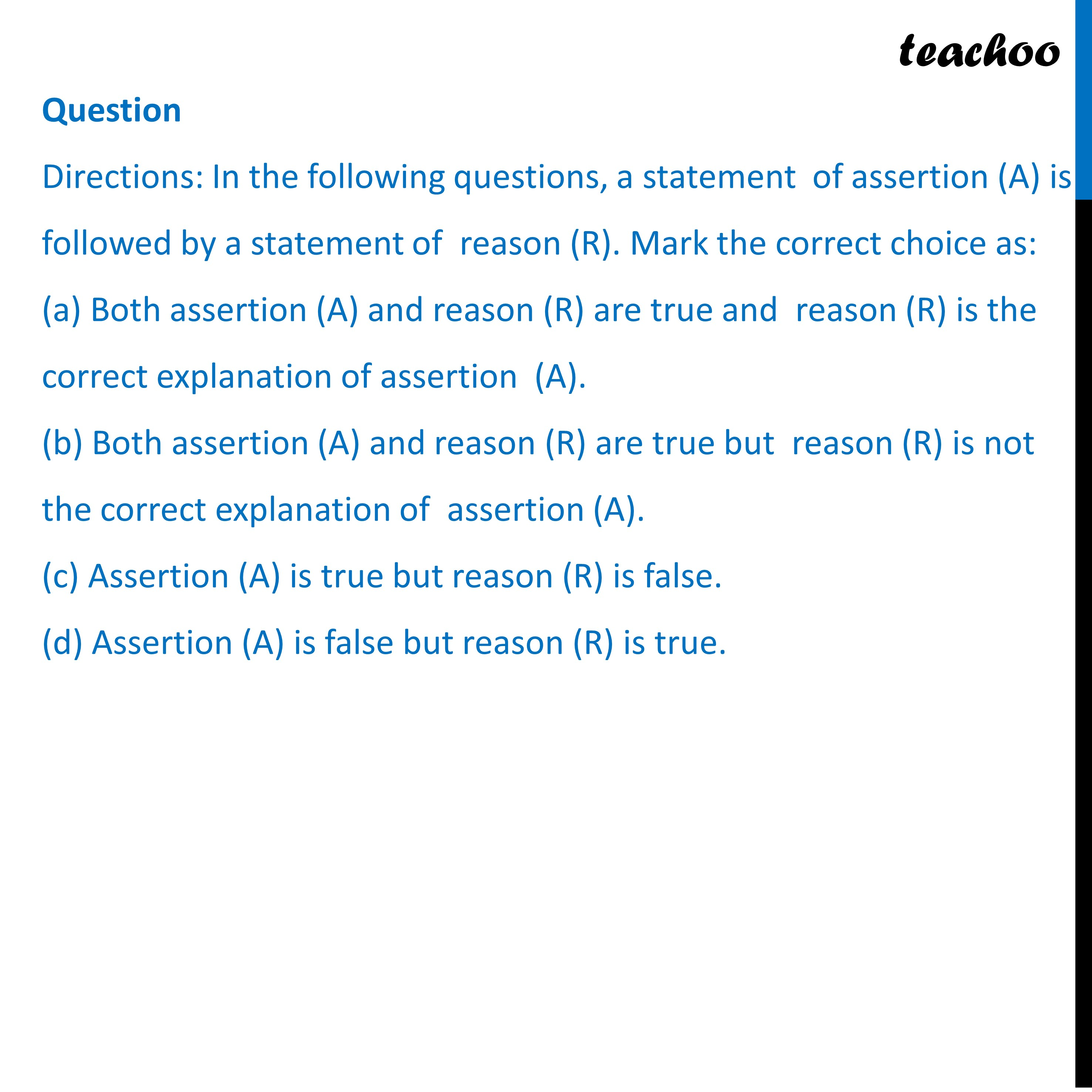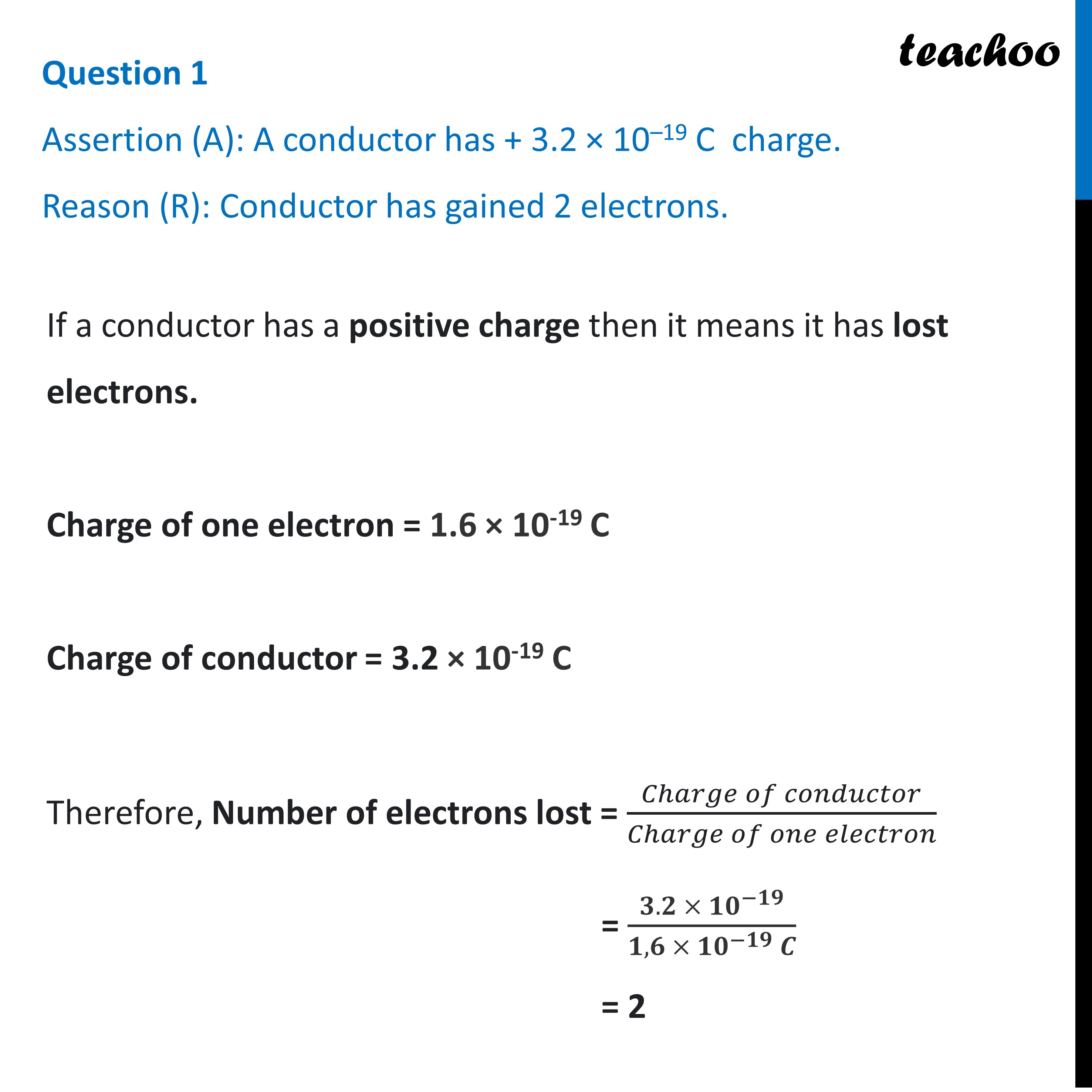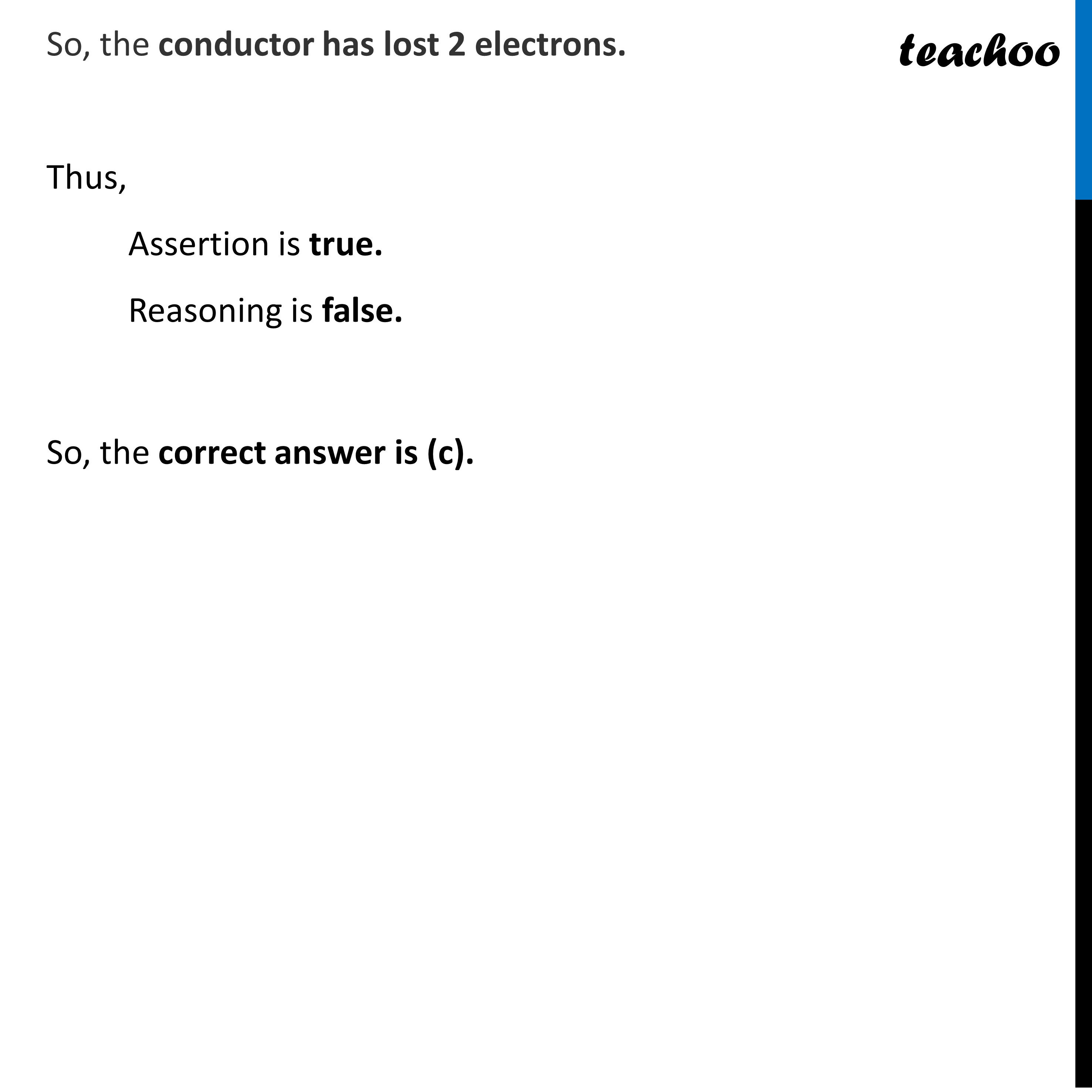Assertion Reasoning questions (MCQ)

Class 10
Chapter 12 Class 10 - Electricity

## Reason (R): Conductor has gained 2 electrons.

If a conductor has a positive charge then it means it has lost electrons.

Charge of one electron = 1.6 × 10 -19 C

Charge of conductor = 3.2 × 10 -19 C

Therefore, Number of electrons lost = Charge of conductor / Charge of one electron

= 3.2 × 10 -19 / 1.6 × 10 -19 C

= 2

So, the conductor has lost 2 electrons.

Thus,

• Assertion is true.
• Reasoning is false.

So, the correct answer is (c).Learn in your speed, with individual attention - Teachoo Maths 1-on-1 Class

### Transcript

Question Directions: In the following questions, a statement of assertion (A) is followed by a statement of reason (R). Mark the correct choice as: (a) Both assertion (A) and reason (R) are true and reason (R) is the correct explanation of assertion (A). (b) Both assertion (A) and reason (R) are true but reason (R) is not the correct explanation of assertion (A). (c) Assertion (A) is true but reason (R) is false. (d) Assertion (A) is false but reason (R) is true. Question 1 Assertion (A): A conductor has + 3.2 × 10–19 C charge. Reason (R): Conductor has gained 2 electrons. If a conductor has a positive charge then it means it has lost electrons. Charge of one electron = 1.6 × 10-19 C Charge of conductor = 3.2 × 10-19 C Therefore, Number of electrons lost = (𝐶ℎ𝑎𝑟𝑔𝑒 𝑜𝑓 𝑐𝑜𝑛𝑑𝑢𝑐𝑡𝑜𝑟)/(𝐶ℎ𝑎𝑟𝑔𝑒 𝑜𝑓 𝑜𝑛𝑒 𝑒𝑙𝑒𝑐𝑡𝑟𝑜𝑛) = (𝟑.𝟐 × 𝟏𝟎^(−𝟏𝟗))/(𝟏,𝟔 × 𝟏𝟎^(−𝟏𝟗) 𝑪) = 2So, the conductor has lost 2 electrons. Thus, Assertion is true. Reasoning is false. So, the correct answer is (c).